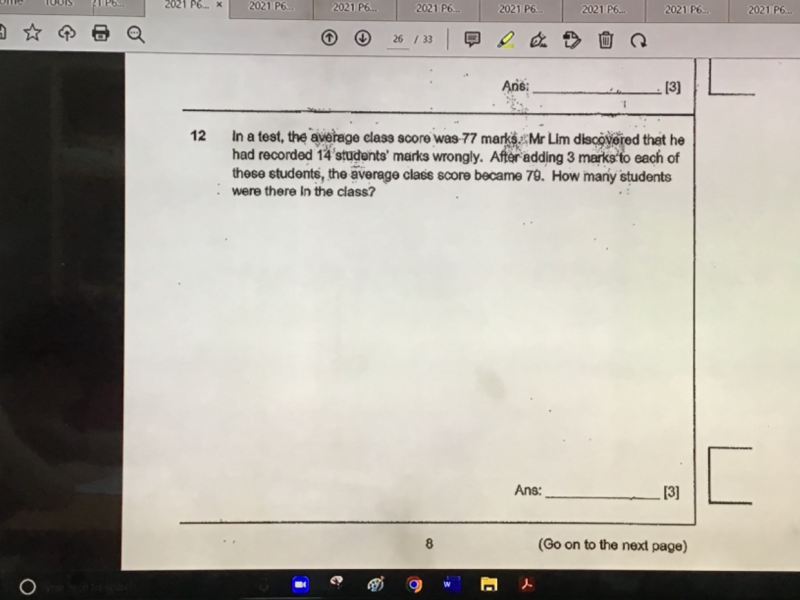# QuestionPls help, thanks

Average = 77

Assume number of students is u

Total = 77u

3 x 14 = 42,  new average =79

79u – 77u = 42

2u = 42   => u = 21

Hence number of students in the class is 21.